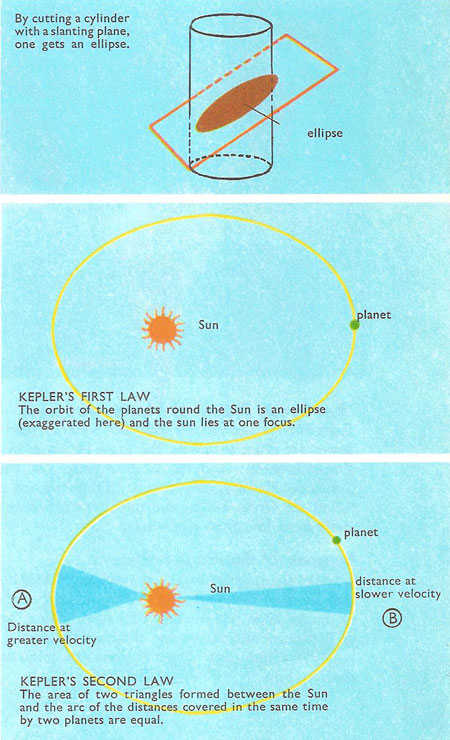A# Kepler's laws of planetary motionKepler's laws are the three basic laws of planetary motion, first published by Johannes Kepler and based on accurate observations by Tycho Brahe. They were subsequently shown by Isaac Newton to be a direct result of his theory of gravitation and laws of motion.

They are:

(1) The elliptical law. Each planet orbits the Sun in an ellipse with the Sun at one focus.

(2) The equal-areas law. A line directed from the Sun to a planet (the radius vector) sweeps out equal areas in equal times as the planet orbits the Sun.

(3) The harmonic law. The square of the period of a planet's orbit varies as the cube of that planet's semi-major axis, the constant of proportionality being the same for all planets.

The first law corrects the simpler model of Copernicus, which assumed circles. The second law expresses the way a planet speeds up when approaching the Sun and slows down when drawing away. The third law gives the exact relation by which planets move faster on orbits which are closer to the Sun.